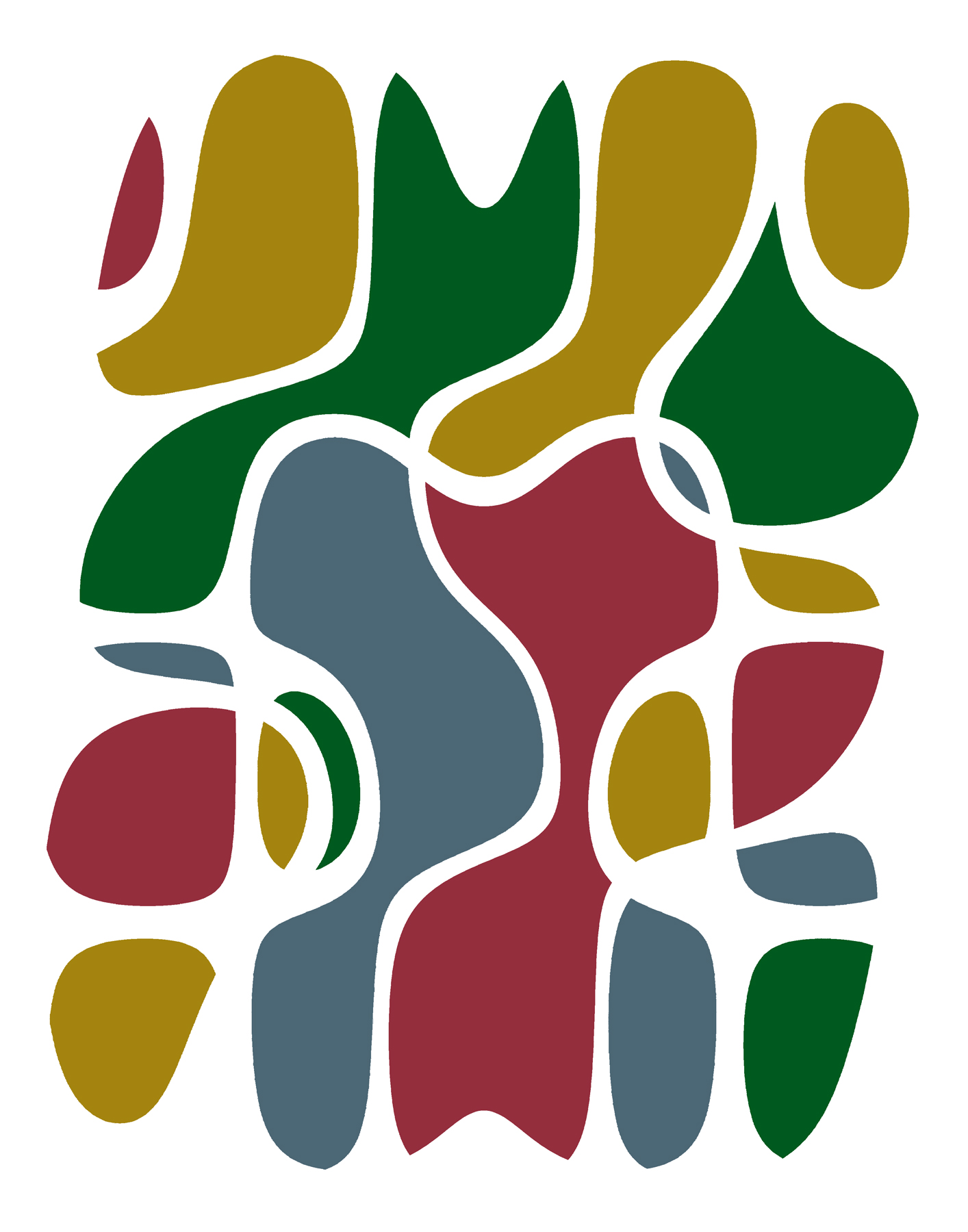# Robert Spann

Washington, DC

I am intrigued by analogs between the compositional rules and color theory principles that artists use and the mathematical/statistical properties of images. For example, equations, just like images, have symmetries. A digital image is a map from the unit square to a set of k colors. Equations with symmetries can be used to produce images with those same symmetries. Currently, I am experimenting with combinations of equations (formed using Discrete Cosine Transforms) that have different symmetries and/or parities. Combining equations with different symmetries can be used to produce images which have structure, but no symmetries. I then refine these images using digital manipulation based on my own aesthetic judgments.Jersey Bounce
50 x 40 cm
Digital Print
2016

I start with two maps, f(x,y) and g(x,y); each a map from the unit square onto the set of integers 1,..,n. Each is constructed using Discrete Cosine Transforms. I combine these two maps to form a map from the unit square onto the integers 1,..,n^2. Each integer is assigned a color. In Jersey Bounce, f(x,y) has color reversing symmetry with respect to a 180 degree rotation. The function g(x,y) is symmetric with respect to a horizontal reflection. The result is an image which has structure, but no symmetries. The initial image is further refined based on my own aesthetic judgments.Flying Horses
50 x 40 cm
Digital Print
2016

I start with two maps, f(x,y) and g(x,y); each a map from the unit square onto the set of integers 1,..,n. Each is constructed using Discrete Cosine Transforms. I combine these two maps to form a map from the unit square onto the integers 1,..,n^2. Each integer is assigned a color. In Flying Horses, f(x,y) is symmetric with respect to a 180 degree rotation. The function g(x,y) is symmetric with respect to a horizontal reflection. The result is an image which has structure, but no symmetries. The initial image is further refined based on my own aesthetic judgments.